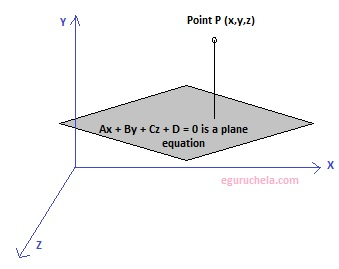### Shortest Distance Between Point and Plane CalculationCalculates the shortest distance in space between given point and a plane equation. The distance from a point to a plane is equal to length of the perpendicular lowered from a point on a plane.
If Ax + By + Cz + D = 0 is a plane equation, then distance from point P(Px , Py , Pz) to plane can be found using the following formula:
The distance from a point to a plane(d) = (APx + BPy + CPz + D)/ √(A+ B2 + C2)
 Please enter the point (coordinates) , , Please enter the equation of the plane x+ y+ z+ =0 Shortest distance between point and plane